## Filters

Sort by :
Clear All
Q

A potential difference of 1V is applied across the other diagonal. What will be the current in the resistor along the diagonal?

1. 0.5 A

2. 1 A

3. 5 A

4. Zero

By closely observing at the figure, you will find that this circuit is a balanced wheat stone bridge. Hence no current will pass through the diagonal. Option (4) is correct
Engineering
64 Views   |

In a large building, there are 15 bulbs of   40 W, 5 bulbs of 100 W, 5 fans of 80 W and 1 heater of 1 kW.  The voltage of the electric mains is 220 V.  The minimum capacity of the main fuse of the building will be :

• Option 1)

8 A

• Option 2)

10 A

• Option 3)

12 A

• Option 4)

14 A

As we have learned

In parallel Grouping -

-

Resistance of each bulb of 40 W

Resistance of 15 bulbs = 1210/15 = 242/3

Resistance of 100 W bulb =

Resistance of 5 such bulbs = 485/5

Resistance of fan =     =  605

Resistance of 5 such fan  = 605 /5

= 121

Resistance of heater =

Equivalent resistance

R = 484 / 25

Option 1)

8 A

Option 2)

10 A

Option 3)

12 A

Option 4)

14 A

View More
Engineering
43 Views   |

In the circuit shown, current (in A) through the 50 V and 30 V batteries are, respectively :• Option 1)

2.5 and 3

• Option 2)

3.5 and 2

• Option 3)

4.5 and 1

• Option 4)

3  and 2.5

As we discussed in

Cell -

The device which converts Chemical energy into electrical energy is known as electric cell.

Option 1)

2.5 and 3

Option 2)

3.5 and 2

Option 3)

4.5 and 1

Option 4)

3  and 2.5

Engineering
45 Views   |

A d.c. main supply of e.m.f. 220 V is connected across a storage battery of e.m.f. 200 V through a resistance of 1. The battery terminals are connected to an external resistance ‘R’. The minimum value of ‘R’, so that a current passes through the battery to charge it is :

• Option 1)• Option 2)• Option 3)• Option 4)

zero

Option 1)Option 2)Option 3)Option 4)

zero

View More
Engineering
111 Views   |In the circuit shown, the current in the 1resistor is :

• Option 1)

1.3 A ,from P to Q

• Option 2)

0 A

• Option 3)

0.13 A ,from Q to P

• Option 4)

0.13 A ,from P to Q

answer is option 3)0.13A from Q  to P

•  Let, Current i1 from 6v battery passes through 3 ohm resistance . At Q current breaks and current i2 goes through 3 ohm and ( i1-i2 ) goes through 1 ohm ,.
• Applying, kirchoffs law in 1st and 2nd loop,we get two eqns as follows
• 4i1-i2=6
• 6i2-i1=9
• solving ,,  i1-i2 =0.13 A
View More
Engineering
83 Views   |

In the electric network shown, when no current flows through theresistor in the arm EB, the potential difference between the points A and D will be :• Option 1)

3V

• Option 2)

4V

• Option 3)

5V

• Option 4)

6V

The potential difference can be calculated via following method:

View More
Engineering
74 Views   |

The supply voltage to a room is 120 V. The resistance of the lead wires is 6. A 60 W bulb is already switched on. What is the decrease of voltage across the bulb, when a 240 W heater is switched on in parallel to the bulb?

• Option 1)

10.04 Volt

• Option 2)

Zero Volt

• Option 3)

2.9 Volt

• Option 4)

13.3 Volt

Need details on this question

Engineering
63 Views   |

Resistance of a given wire is obtained  by measuring the current flowing in it and the voltage difference applied across it. If the percentage errors in the measurement of the current and the voltage difference are 3% each, then error in the value of resistance of the wire is

• Option 1)

6%

• Option 2)

zero

• Option 3)

1%

• Option 4)

3%

As discussed in

Ohm's Law -

Current flowing through the conductor is directly proportional to the Potential difference accross two ends .

- wherein

unit -

Electric Resistance

Percentage error in is

Option 1)

6%

This option is correct.

Option 2)

zero

This option is incorrect.

Option 3)

1%

This option is incorrect.

Option 4)

3%

This option is incorrect.

View More
Engineering
65 Views   |

This question has Statement I and Statement II. Of the four choices given after the Statements, choose the one that best describes the two Statemens.

Statement - I : Higher the range, greater is the resistance of ammeter.

Statement - II : To increase the range of ammeter,additional shunt needs to be used across it.

• Option 1)

Statement - I is false, Statement - II is true.

• Option 2)

Statement - I is true, Statement - II is true, Statement - II is the correct explanation of Statement - I.

• Option 3)

Statement - I is true, Statement - II is true, Statement - II is not the correct explanation of Statement - I.

• Option 4)

Statement - I is true, Statement - II is false.

As discussed in-

Required shunt -

- wherein

Current through galvanometer

The answer follows from basic Principle of stunt and its use to make an ammeter:

Option 1)

Statement - I is false, Statement - II is true.

This option is correct.

Option 2)

Statement - I is true, Statement - II is true, Statement - II is the correct explanation of Statement - I.

This option is incorrect.

Option 3)

Statement - I is true, Statement - II is true, Statement - II is not the correct explanation of Statement - I.

This option is incorrect.

Option 4)

Statement - I is true, Statement - II is false.

This option is incorrect.

View More
Engineering
77 Views   |

A 10 V battery with internal resistanceand a 15V battery with internal resistanceare connected in parallel to a voltmeter (see figure). The reading in the voltmeter will be close to :• Option 1)

11.9 V

• Option 2)

12.5 V

• Option 3)

13.1 V

• Option 4)

24.5 V

As we discussed in

In closed loop -

- wherein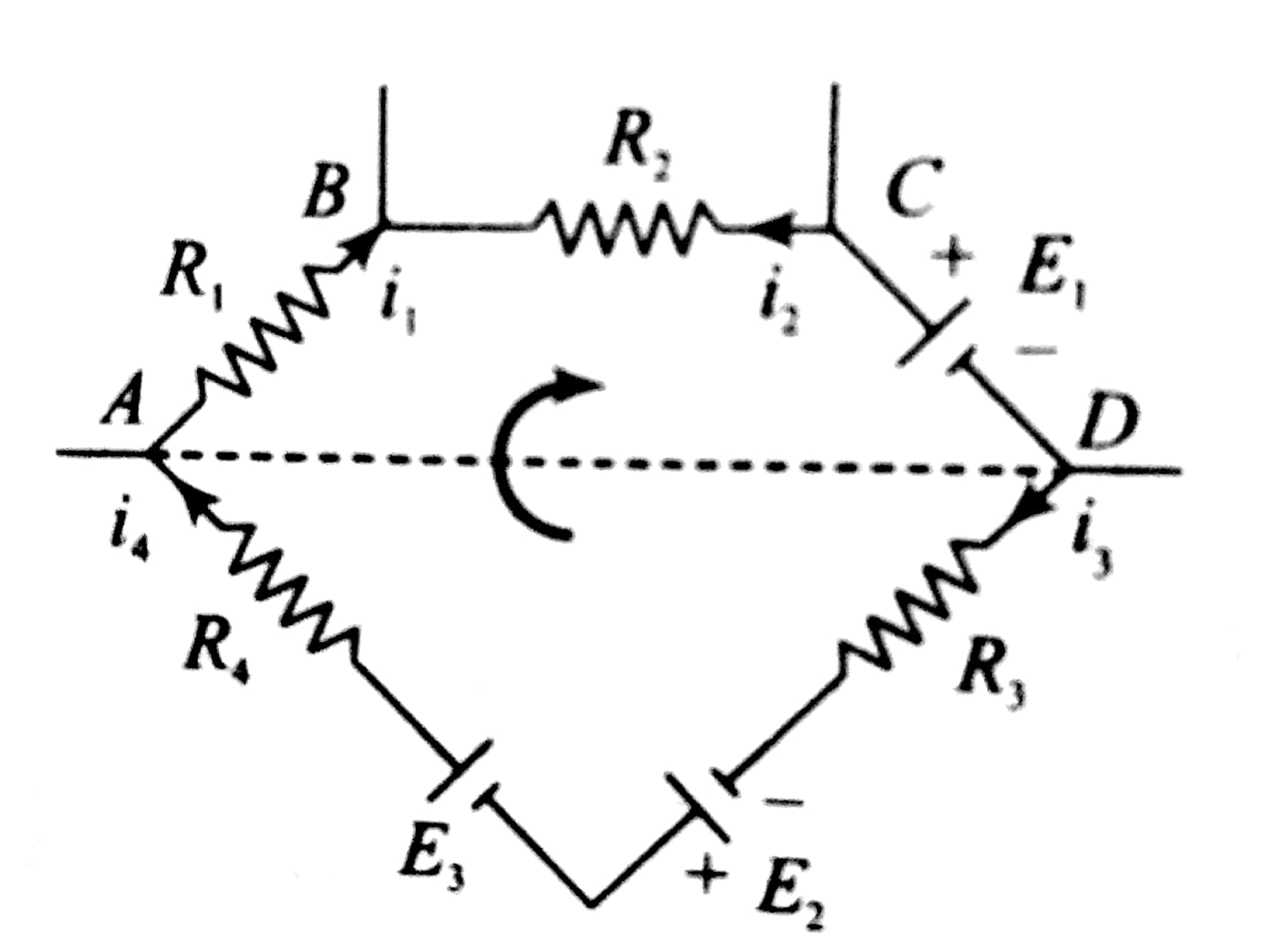Current in the circuit

Option 1)

11.9 V

The option is incorrect

Option 2)

12.5 V

The option is incorrect

Option 3)

13.1 V

The option is correct

Option 4)

24.5 V

The option is incorrect

View More
Engineering
27 Views   |

A 3 olt battery with  negligible internal resistance is connected in a circuit as shown in the figure. The current I i n the circuit will be• Option 1)• Option 2)• Option 3)• Option 4)as discussed in

Parallel Grouping -

Potential - Same

Current - Different

- wherein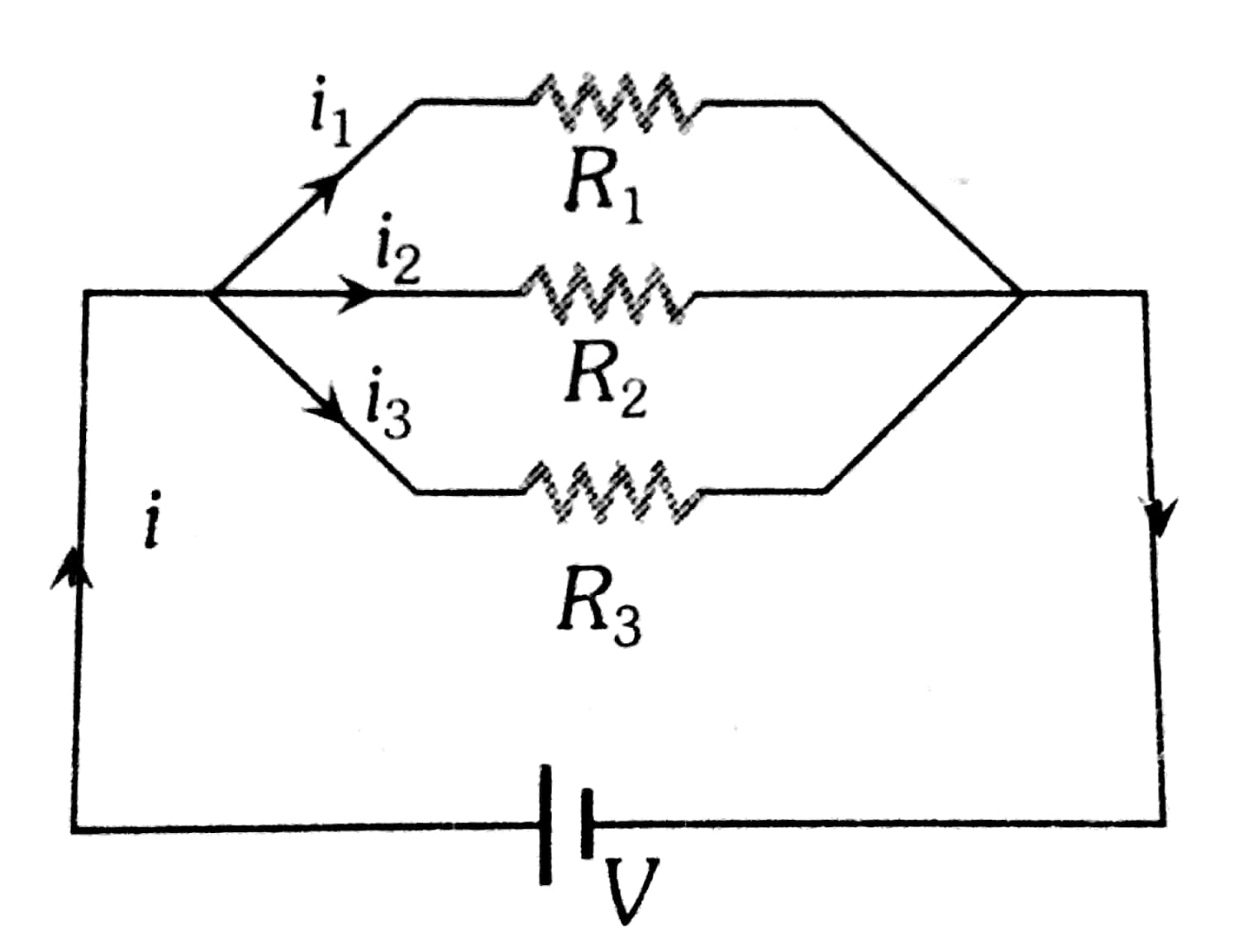Option 1)This option is incorrect.

Option 2)This option is correct.

Option 3)This option is incorrect.

Option 4)This option is incorrect.

View More
Engineering
32 Views   |

Two electric bulbs marked  25 W-220 V and 100 W - 220 V are connected in series to a 440 V supply .Which of the bulbs will fuse?

• Option 1)

both

• Option 2)

100W

• Option 3)

25 W

• Option 4)

neither

As discussed in:

Series Grouping -

Potential - Different

Current - Same

- wherein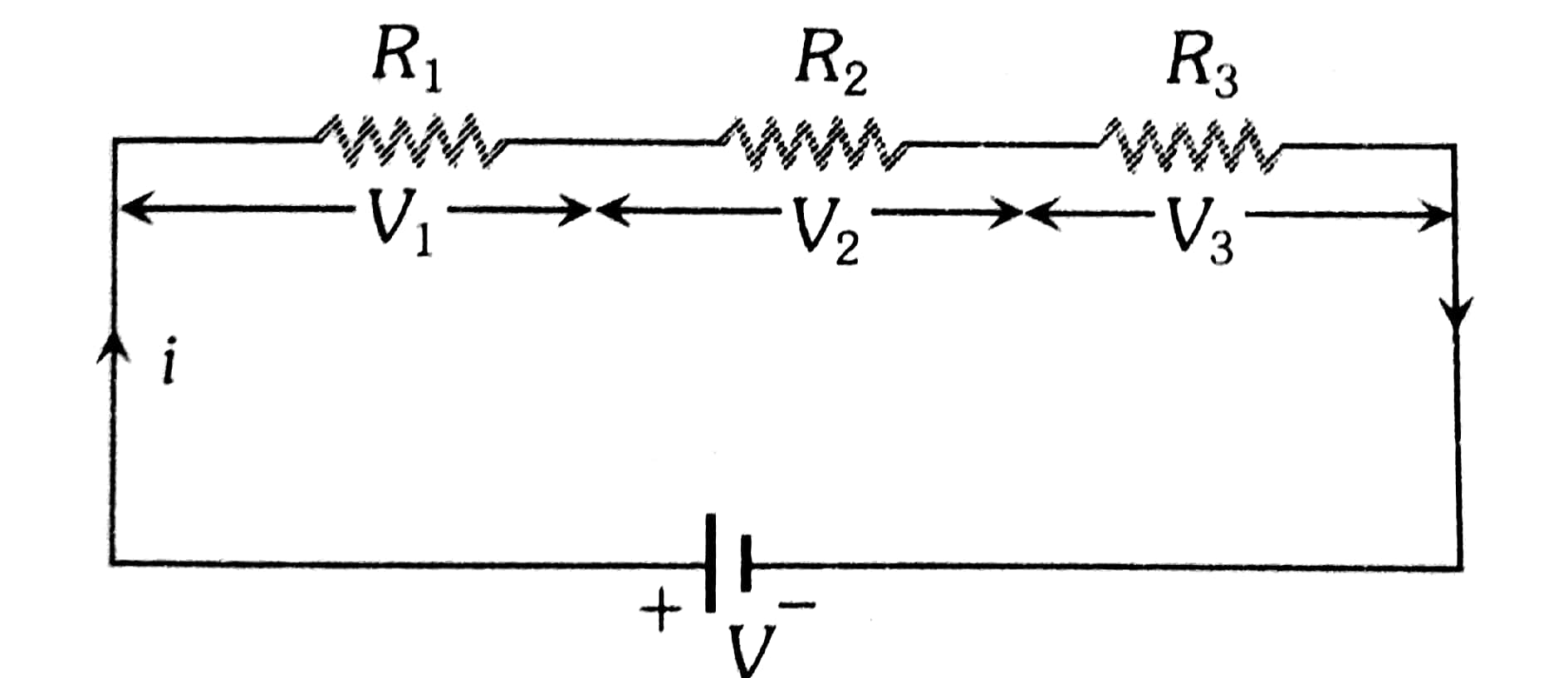Similarly,

The current flowing through the circuit

After solving this, we get

Thus the bulb rated 25W-220V will fuse.

Option 1)

both

Option 2)

100W

Option 3)

25 W

Option 4)

neither

View More
Engineering
125 Views   |

The circuit shown here has two batteries of 8.0 V and 16.0 V and three resistors 3, 9and 9and a capacitor 5.0F.How much is the current I in the circuit in steady state ?

• Option 1)

1.6 A

• Option 2)

0.67 A

• Option 3)

2.5 A

• Option 4)

0.25 A

As we have learned

In closed loop -

- whereinsince in steady state current through capacitor is  0

USing kirchoff's rule

16-9I -3I -8V = 0   or I = 8/12 A = 2/3 A

= 0.67 A

Option 1)

1.6 A

Option 2)

0.67 A

Option 3)

2.5 A

Option 4)

0.25 A

View More
Engineering
179 Views   |

Four identical electrical lamps are labelled 1.5V, 0.5A which describes the condition necessary for them to operate at normal brightness. A 12V battery of negligible internal resistance is connected to lamps as shown, then• Option 1)

The value of R for normal brightness of each lamp is

• Option 2)

The value of R for normal brightness of each lamp is

• Option 3)

Total power dissipated in circuit when all lamps are normally bright is 24W

• Option 4)

Power dissipated in R is 21W when all lamps are normally bright

V = IR    For normal brightness current through each bulb is 0.5 A Therefore, Total current = 2A Option 1) The value of R for normal brightness of each lamp is   Incorrect Option 2) The value of R for normal brightness of each lamp is Correct Option 3) Total power dissipated in circuit when all lamps are normally bright is 24W Incorrect Option 4) Power dissipated in R is 21W when all lamps...
Engineering
617 Views   |

When the rms voltages VL, VC and VR are  measured respectively across the inductor L, the capacitor C and the resistor R in a series  LCR  circuit  connected  to  an AC source, it is found that the ratio VL : VC : VR = 1 : 2 : 3. If the rms voltage of the AC source is 100 V, then VR is close to :

• Option 1)

50 V

• Option 2)

70 V

• Option 3)

90 V

• Option 4)

100 V

or   Option 1) 50 V Incorrect Option 2) 70 V Incorrect Option 3) 90 V Correct Option 4) 100 V Incorrect
Engineering
939 Views   |

Water boils in an electric kettle in 15 minutes after switching on. If the length of the heating wire is decreased to 2/3 of its initial value, then the same amount of water will boil with the same supply voltage in

• Option 1)

15 minutes

• Option 2)

12 minutes

• Option 3)

10 minutes

• Option 4)

8 minutes

As we learnt in  Resistance formula - - wherein  resistivity of material  Number of free electrons per unit volume.   Initial power   Final power  Since energy is same: Pi ti = Pf tf   Option 1) 15 minutes This solution is incorrect Option 2) 12 minutes This solution is incorrect Option 3) 10 minutes This solution is correct Option 4) 8 minutes This solution is incorrect
Engineering
239 Views   |

The three resistance of equal value are arranged in the different combinations shown below. Arrange them in increasing order of power dissipation

I.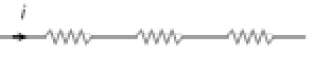II.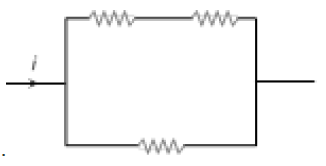III.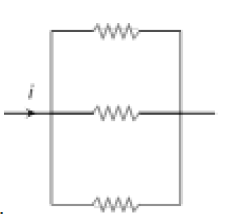IV.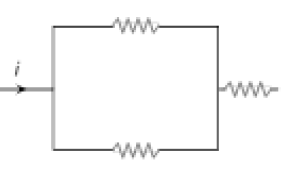• Option 1)

III < II < IV < I

• Option 2)

II < III < IV < I

• Option 3)

I < IV < III < II

• Option 4)

I < III < II < IV

As we learnt in  Series Grouping - Potential - Different Current - Same - wherein    & Parallel Grouping - Potential - Same Current - Different - wherein       P is maximum when R is     Order of power  iii <  ii < iv < i Option 1) III < II < IV < I This solution is correct Option 2) II < III < IV < I This solution is incorrect Option 3) I < IV < III < II This solution is incorrect Option...
Engineering
226 Views   |

The resistance of the filament of an electric bulb changes with temperature. If an electric bulb rated 220 volt and 100 watt is connected to (220×.8) volt sources, then the actual power would be

• Option 1)

100×0.8 watt

• Option 2)

100× (0.8)2 watt

• Option 3)

Between 100×0.8 watt and 100 watt

• Option 4)

Between 100× (0.8)2 watt and 100×0.8 watt

As we learnt in  Power dissipiated in external resistance - -     Applied Voltage=  maximum power =  Option 1) 100×0.8 watt This solution is incorrect Option 2) 100× (0.8)2 watt This solution is incorrect Option 3) Between 100×0.8 watt and 100 watt This solution is incorrect Option 4) Between 100× (0.8)2 watt and 100×0.8 watt This solution is correct
Engineering
683 Views   |

In the circuit shown in figure, the heat produced in 5 ohm resistance is 10 calories per second. The heat produced in 4 resistance is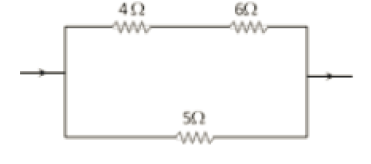• Option 1)

1 cal/sec

• Option 2)

2 cal/sec

• Option 3)

3 cal/sec

• Option 4)

4 cal/sec

As we learnt in Current through any resistance - - wherein   Let current through  be I1 and current through  be I2 . Then,  Option 1) 1 cal/sec This option is incorrect Option 2) 2 cal/sec This option is correct Option 3) 3 cal/sec This option is incorrect Option 4) 4 cal/sec This option is incorrect
Engineering
338 Views   |

In the circuit as shown in the figure, the heat produced by 6 ohm resistance due to current flowing in it is 60 calorie per second. The heat generated across 3 ohm resistance per second will be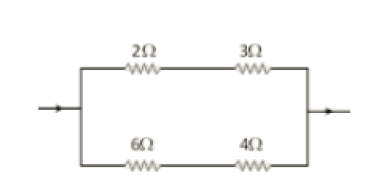• Option 1)

30 cal

• Option 2)

60 cal

• Option 3)

120 cal

• Option 4)

100 cal

As we learnt in  Power dissipiated in external resistance - -                                               Rate of Heat production          Option 1) 30 cal Incorrect Option 2) 60 cal Incorrect Option 3) 120 cal correct Option 4) 100 cal Incorrect
Exams
Articles
Questions